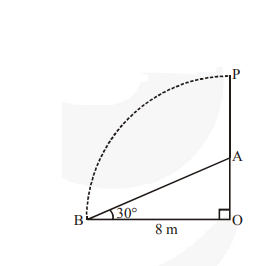# A tree breaks due to storm and the broken part bends so that the top of the trees touches the ground making an angle of 30° with the ground.Question:

A tree breaks due to storm and the broken part bends so that the top of the trees touches the ground making an angle of 30° with the ground. The distance between the foot of the tree to the point where the top touches the ground is 8 m. Find the height of the tree.

Solution:

Let tree is broken at A and its top is touching the ground at B.

Now, in right $\triangle \mathrm{AOB}$, we have$\frac{\mathbf{A O}}{\mathbf{O B}}=\tan 30^{\circ}$

$\Rightarrow \frac{\mathbf{A 0}}{\mathbf{8}}=\frac{1}{\sqrt{\mathbf{3}}}$

$\Rightarrow \frac{\mathbf{A 0}}{\mathbf{8}}=\frac{1}{\sqrt{\mathbf{3}}} \Rightarrow \mathrm{AO}=\frac{\mathbf{8}}{\sqrt{\mathbf{3}}} \mathrm{m}$

Also, $\frac{\mathbf{A B}}{\mathbf{O B}}=\sec 30^{\circ}$

$\Rightarrow \frac{\mathbf{A B}}{\mathbf{8}}=\frac{\mathbf{2}}{\sqrt{\mathbf{3}}}$

$\mathrm{AB}=\frac{\mathbf{2} \times \mathbf{8}}{\sqrt{\mathbf{3}}}=\frac{\mathbf{1 6}}{\sqrt{\mathbf{3}}} \mathrm{m}$

Now, height of the tree $\mathrm{OP}=\mathrm{OA}+\mathrm{AB}$

$=\frac{8}{\sqrt{3}}+\frac{16}{\sqrt{3}}=\frac{24}{\sqrt{3}} \times \frac{\sqrt{3}}{\sqrt{3}} m=8 \sqrt{3} m$